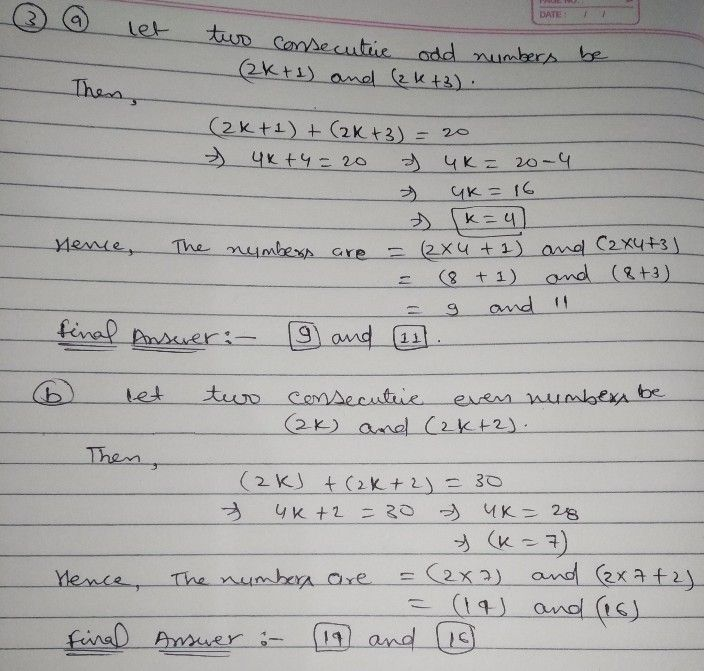Symbol
Problem$3$ $\right)$ ) If the sum of two consecutive odd numbers is $20$ find the numbers. b) If the sum of two consecutive even numbers is $3\left(\right)$ find the numbers. $x$